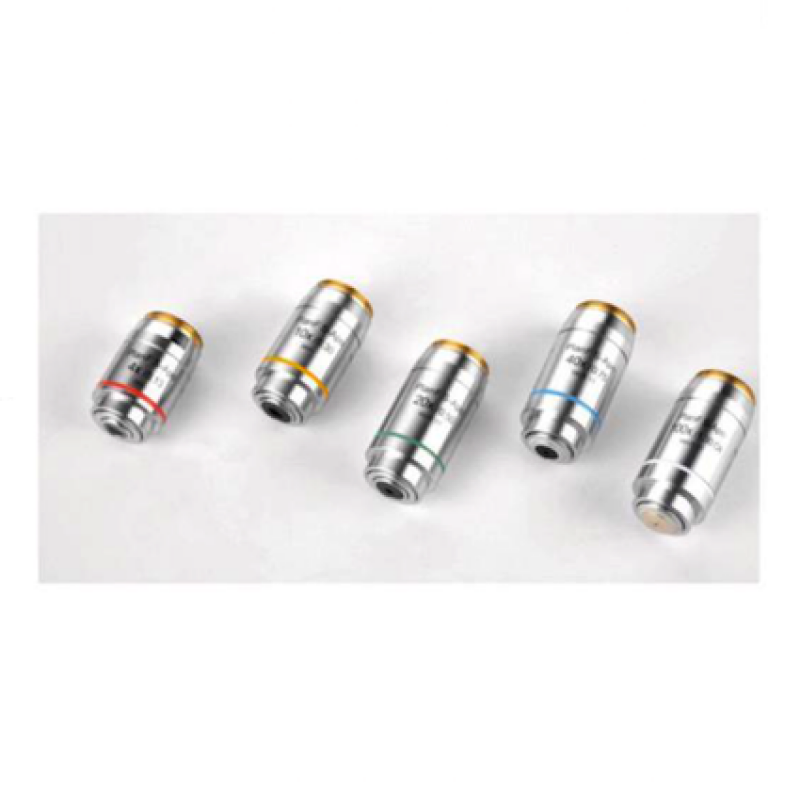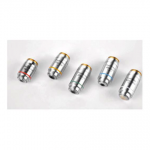•• 简介-4X NA=0.13 WD=16.5 -10X NA=0.3 WD=8.1 -20X NA=0.5 WD=2.1 -40X（弹簧） NA=0.75 WD=0.7 -100X（弹簧、油） NA=1.3 WD=0.15-5X半复消色差金相物镜 NA=0.15 WD=20 明暗场 -10X半复消色差金相物镜 NA=0.3 WD=11 明暗场 -20X半复消色差金相物镜 NA=0.45 WD=3.1 明暗场 -50X（弹簧）复消色差金相物镜 NA=0.8 WD=1 明暗场 -100X（弹簧、干）复消色差金相物镜 NA=0.9 WD=1 明暗场

### 相关产品

QQ 咨询 邮件咨询